Anúncio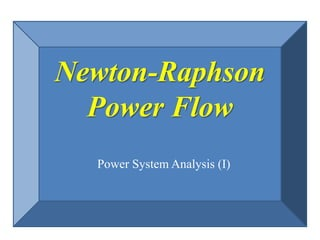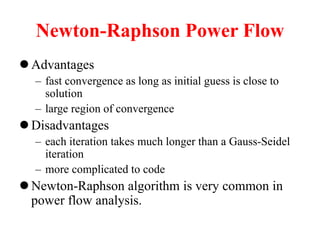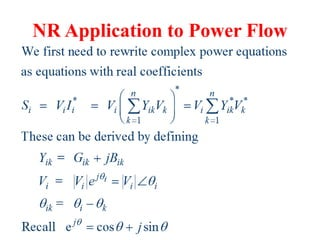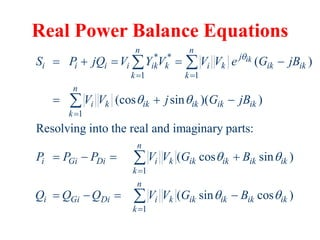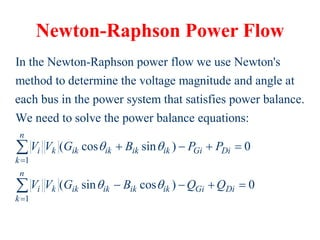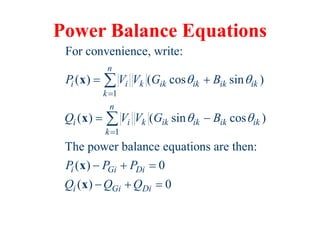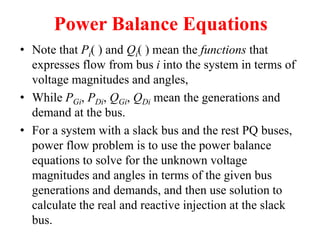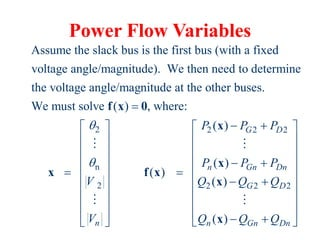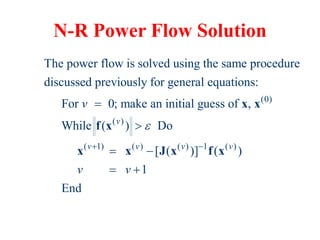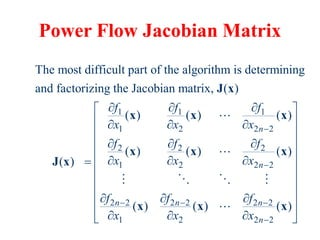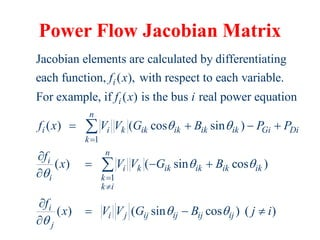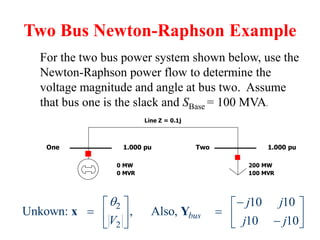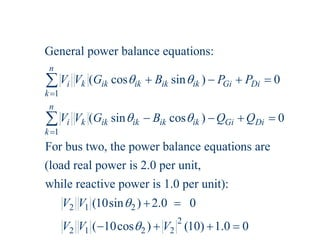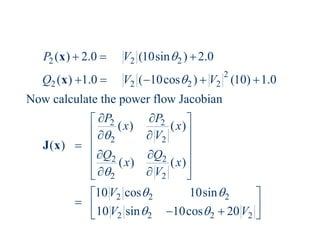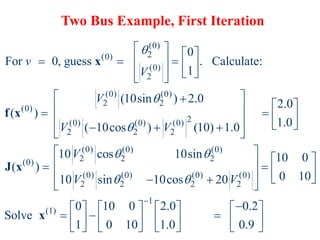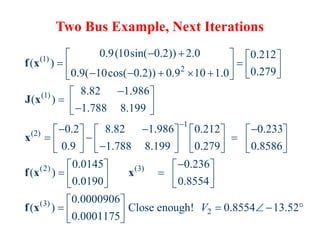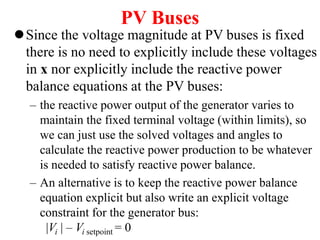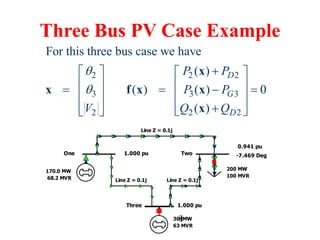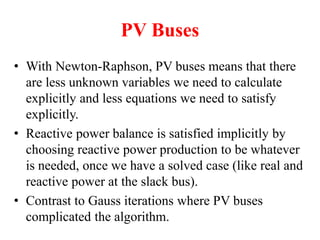1 de 19
Anúncio

### NR-Power Flow.pdf

1. Newton-Raphson Power Flow Power System Analysis (I)
2. Newton-Raphson Power Flow  Advantages – fast convergence as long as initial guess is close to solution – large region of convergence  Disadvantages – each iteration takes much longer than a Gauss-Seidel iteration – more complicated to code  Newton-Raphson algorithm is very common in power flow analysis.
3. NR Application to Power Flow
4. Real Power Balance Equations * * 1 1 1 1 1 ( ) (cos sin )( ) Resolving into the real and imaginary parts: ( cos sin ) ( sin ik n n j i i i i ik k i k ik ik k k n i k ik ik ik ik k n i Gi Di i k ik ik ik ik k n i Gi Di i k ik ik k S P jQ V Y V V V e G jB V V j G jB P P P V V G B Q Q Q V V G                                cos ) ik ik B  
5. Newton-Raphson Power Flow In the Newton-Raphson power flow we use Newton's method to determine the voltage magnitude and angle at each bus in the power system that satisfies power balance. We need to solve the power balance equ 1 1 ations: ( cos sin ) 0 ( sin cos ) 0 n i k ik ik ik ik Gi Di k n i k ik ik ik ik Gi Di k V V G B P P V V G B Q Q                
6. Power Balance Equations 1 1 For convenience, write: ( ) ( cos sin ) ( ) ( sin cos ) The power balance equations are then: ( ) 0 ( ) 0 n i i k ik ik ik ik k n i i k ik ik ik ik k i Gi Di i Gi Di P V V G B Q V V G B P P P Q Q Q                   x x x x
7. Power Balance Equations • Note that Pi( ) and Qi( ) mean the functions that expresses flow from bus i into the system in terms of voltage magnitudes and angles, • While PGi, PDi, QGi, QDi mean the generations and demand at the bus. • For a system with a slack bus and the rest PQ buses, power flow problem is to use the power balance equations to solve for the unknown voltage magnitudes and angles in terms of the given bus generations and demands, and then use solution to calculate the real and reactive injection at the slack bus.
8. Power Flow Variables 2 n 2 Assume the slack bus is the first bus (with a fixed voltage angle/magnitude). We then need to determine the voltage angle/magnitude at the other buses. We must solve ( ) , where: n V V          f x 0 x 2 2 2 2 2 2 ( ) ( ) ( ) ( ) ( ) G D n Gn Dn G D n Gn Dn P P P P P P Q Q Q Q Q Q                                         x x f x x x
9. N-R Power Flow Solution (0) ( ) ( 1) ( ) ( ) 1 ( ) The power flow is solved using the same procedure discussed previously for general equations: For 0; make an initial guess of , While ( ) Do [ ( )] ( ) 1 End v v v v v v v v          x x f x x x J x f x
10. Power Flow Jacobian Matrix 1 1 1 1 2 2 2 2 2 2 1 2 2 2 2 2 2 2 2 2 1 2 The most difficult part of the algorithm is determining and factorizing the Jacobian matrix, ( ) ( ) ( ) ( ) ( ) ( ) ( ) ( ) ( ) ( ) n n n n n f f f x x x f f f x x x f f f x x x                         J x x x x x x x J x x x 2 2 ( ) n                     x
11. Power Flow Jacobian Matrix 1 Jacobian elements are calculated by differentiating each function, ( ), with respect to each variable. For example, if ( ) is the bus real power equation ( ) ( cos sin ) i i n i i k ik ik ik ik Gi k f x f x i f x V V G B P P         1 ( ) ( sin cos ) ( ) ( sin cos ) ( ) Di n i i k ik ik ik ik i k k i i i j ij ij ij ij j f x V V G B f x V V G B j i                   
12. Two Bus Newton-Raphson Example Line Z = 0.1j One Two 1.000 pu 1.000 pu 200 MW 100 MVR 0 MW 0 MVR For the two bus power system shown below, use the Newton-Raphson power flow to determine the voltage magnitude and angle at bus two. Assume that bus one is the slack and SBase = 100 MVA. 2 2 10 10 Unkown: , Also, 10 10 bus j j V j j                  x Y
13. 1 1 General power balance equations: ( cos sin ) 0 ( sin cos ) 0 For bus two, the power balance equations are (load real power is 2.0 per unit, while react n i k ik ik ik ik Gi Di k n i k ik ik ik ik Gi Di k V V G B P P V V G B Q Q                 2 1 2 2 2 1 2 2 ive power is 1.0 per unit): (10sin ) 2.0 0 ( 10cos ) (10) 1.0 0 V V V V V        
14. 2 2 2 2 2 2 2 2 2 2 2 2 2 2 2 2 2 2 2 2 2 2 2 ( ) 2.0 (10sin ) 2.0 ( ) 1.0 ( 10cos ) (10) 1.0 Now calculate the power flow Jacobian ( ) ( ) ( ) ( ) ( ) 10 cos 10sin 10 sin 10cos 20 P V Q V V P P x x V Q Q x x V V V V                                               x x J x
15. Two Bus Example, First Iteration (0) 2 (0) (0) 2 (0) (0) 2 2 (0) 2 (0) (0) (0) 2 2 2 (0) (0) (0) 2 2 2 (0) (0) (0) 2 2 0 For 0, guess . Calculate: 1 (10sin ) 2.0 2.0 ( ) 1.0 ( 10cos ) (10) 1.0 10 cos 10sin ( ) 10 sin 10cos v V V V V V V                                              x f x J x (0) (0) 2 2 1 (1) 10 0 0 10 20 0 10 0 2.0 0.2 Solve 1 0 10 1.0 0.9 V                                               x
16. Two Bus Example, Next Iterations (1) 2 (1) 1 (2) 0.9(10sin( 0.2)) 2.0 0.212 ( ) 0.279 0.9( 10cos( 0.2)) 0.9 10 1.0 8.82 1.986 ( ) 1.788 8.199 0.2 8.82 1.986 0.212 0.233 0.9 1.788 8.199 0.279 0.8586 (                                                               f x J x x f (2) (3) (3) 2 0.0145 0.236 ) 0.0190 0.8554 0.0000906 ( ) Close enough! 0.8554 13.52 0.0001175 V                           x x f x
17. PV Buses Since the voltage magnitude at PV buses is fixed there is no need to explicitly include these voltages in x nor explicitly include the reactive power balance equations at the PV buses: – the reactive power output of the generator varies to maintain the fixed terminal voltage (within limits), so we can just use the solved voltages and angles to calculate the reactive power production to be whatever is needed to satisfy reactive power balance. – An alternative is to keep the reactive power balance equation explicit but also write an explicit voltage constraint for the generator bus: |Vi | – Vi setpoint = 0
18. Three Bus PV Case Example Line Z = 0.1j Line Z = 0.1j Line Z = 0.1j One Two 1.000 pu 0.941 pu 200 MW 100 MVR 170.0 MW 68.2 MVR -7.469 Deg Three 1.000 pu 30 MW 63 MVR 2 2 2 3 3 3 2 2 2 For this three bus case we have ( ) ( ) ( ) 0 ( ) D G D P P P P V Q Q                             x x f x x x
19. PV Buses • With Newton-Raphson, PV buses means that there are less unknown variables we need to calculate explicitly and less equations we need to satisfy explicitly. • Reactive power balance is satisfied implicitly by choosing reactive power production to be whatever is needed, once we have a solved case (like real and reactive power at the slack bus). • Contrast to Gauss iterations where PV buses complicated the algorithm.
Anúncio